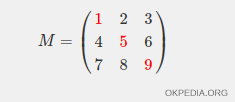# How to calculate trace of a matrix in Matlab/Octave

To determine the trace (linear algebra) of a matrix in Matlab/Octave use the function trace()

trace(M)

The parameter M is the name of the matrix

The function trace() calculates the trace of the matrix.

What is a matrix trace? It is the algebraic sum of the elements on its main diagonal. The main diagonal starts at the top left and ends at the bottom right. For example, in the following matrix are the red elements 1, 5, 9. So, the trace of the matrix is the sum 1 + 5 + 9 = 15.## Example

Create a 3x3 square matrix with three rows and three columns

>> M=[1 2 3; 4 5 6; 7 8 9]
M =
1 2 3
4 5 6
7 8 9

The main diagonal of the matrix is made up of the elements {1,5,9}.

Calculate the trace using the function trace()

>> trace(M)
ans = 15

The trace of the matrix is equal to 15, because the algebraic sum of the elements in the main diagonal is 1 + 5 + 9 = 15.

https://how.okpedia.org/en/matlab/how-to-calculate-trace-of-a-matrix-in-matlaboctaveReport us an error or send a suggestion to improve this page

Matrix in Matlab/Octave